/

### Marginal Tail Value-at-Risk (Marginal TVaR)

Suppose we have a set ofrisk factors which we can characterise by an-dimensional vector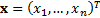. Suppose that the (active) exposures we have to these factors are characterised by another-dimensional vector,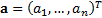. Then the aggregate exposure is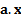.

The Value-at-Risk of the portfolio of exposures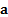at confidence level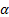,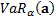, is usually defined to be the value such that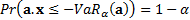.

The Tail Value-at-Risk is defined as: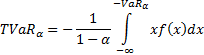The Marginal Tail Value-at-Risk,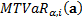, is the sensitivity of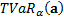to a small change in’th exposure. It is therefore: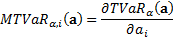Because Tail Value-at-Risk is (first-order) homogeneous (for a continuous probability distribution) it satisfies the Euler capital allocation principle and hence: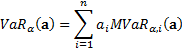If the risk factors are multivariate normally distributed then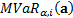can be expressed using a relatively simple formula, see here.